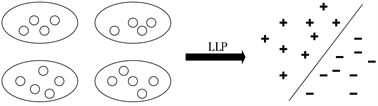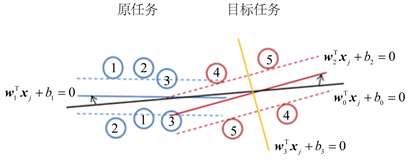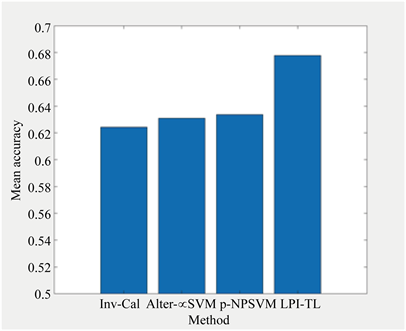# 一种基于标签比例信息的迁移学习算法A Label Proportion Information-Based Transfer Learning Algorithm

DOI: 10.12677/CSA.2020.102035, PDF, 下载: 225  浏览: 530  国家自然科学基金支持

Abstract: The learning with label proportions problem is a learning task that only uses bag’s label propor-tions information to build a classification model. Due to insufficient training samples, the existing methods that viewed the above problem as single task did not perform well in text classification. To some extent, transfer learning can solve the problem of insufficient training data, the problem that how to use historical data (the original task data) to help the newly generated data (target task data) to classify becomes extremely important. This paper presents a label proportion information-based transfer learning approach to transfer knowledge from the source task to the target task, helping the target task to build a classifier. In order to obtain the transfer learning model, this method converted the original optimization problem into a convex optimization problem, and then solved the dual optimization problem to establish an accurate classifier for the target task. Extensive experiments have shown that the proposed method outperforms the traditional methods.

1. 引言

1) 结合支持向量回归算法提出了基于标签比例信息的迁移学习模型，该模型可以利用迁移学习将知识从原任务迁移到目标任务。

2) 利用拉格朗日方法将原始目标模型转换为凸优化问题，并获得原任务和目标任务的预测模型。

3) 在多个数据集上进行广泛实验，并与现有算法进行对比，验证了提出算法的有效性。

2. 问题描述与相关工作

2.1. 问题描述Figure 1. Two-class label proportions learning problem

2.2. 相关工作

3. 标签比例学习算法

$y=-\mathrm{log}\left(\frac{1}{p}-1\right)$ (1)

${\forall }_{i}:\frac{1}{|{B}_{i}|}\underset{j\in {B}_{i}}{\sum }\left({w}^{\text{T}}{x}_{j}+b\right)={y}_{i}$(2)

3.1. 目标函数

${f}_{1}\left(x\right)={w}_{1}^{\text{T}}\cdot x+{b}_{1}$ (3)

${f}_{2}\left(x\right)={w}_{2}^{\text{T}}\cdot x+{b}_{2}$ (4)

$\mathrm{min}\text{ }\frac{1}{2}{‖{w}_{0}‖}^{2}+\frac{{\lambda }_{1}}{2}{‖{v}_{1}‖}^{2}+\frac{{\lambda }_{2}}{2}{‖{v}_{2}‖}^{2}+{C}_{1}\underset{i=1}{\overset{{t}_{1}}{\sum }}\left({\xi }_{1i}+{\xi }_{1i}^{*}\right)+{C}_{2}\underset{m=1}{\overset{{t}_{2}}{\sum }}\left({\xi }_{2m}+{\xi }_{2m}^{*}\right)$ (5)

${\forall }_{i=1}^{{t}_{1}}:\begin{array}{l}\frac{1}{|{B}_{i}^{s}|}\underset{j\in {B}_{i}^{s}}{\sum }\left({w}_{1}^{\text{T}}{x}_{j}+{b}_{1}\right)-{y}_{i}\le {\epsilon }_{1i}+{\xi }_{1i}\hfill \\ {y}_{i}-\frac{1}{|{B}_{i}^{s}|}\underset{j\in {B}_{i}^{s}}{\sum }\left({w}_{1}^{\text{T}}{x}_{j}+{b}_{1}\right)\le {\epsilon }_{1i}+{\xi }_{1i}^{*}\hfill \end{array}$

${\forall }_{m=1}^{{t}_{2}}:\begin{array}{l}\frac{1}{|{B}_{m}^{t}|}\underset{n\in {B}_{m}^{t}}{\sum }\underset{n\in {B}_{m}^{t}}{\sum }\left({w}_{2}^{\text{T}}{x}_{n}+{b}_{2}\right)-{y}_{m}\le {\epsilon }_{2m}+{\xi }_{2m}\hfill \\ {y}_{m}-\frac{1}{|{B}_{m}^{t}|}\underset{n\in {B}_{m}^{t}}{\sum }\left({w}_{2}^{\text{T}}{x}_{n}+{b}_{2}\right)\le {\epsilon }_{2m}+{\xi }_{2m}^{*}\hfill \end{array}$

${\xi }_{1n},{\xi }_{1n}^{*}\ge 0\text{\hspace{0.17em}}\left(n=1,\cdots ,{t}_{1}\right)$

${\xi }_{2m},{\xi }_{2m}^{*}\ge 0\text{\hspace{0.17em}}\left(m=1,\cdots ,{t}_{2}\right)$Figure 2. Transfer knowledge from the source task to the target task

3.2. 对偶问题

$\begin{array}{l}\frac{1+{\lambda }_{1}}{2{\lambda }_{1}}\underset{i,j=1}{\overset{{t}_{1}}{\sum }}\frac{\left({\alpha }_{1i}^{*}-{\alpha }_{1i}\right)\left({\alpha }_{1j}^{*}-{\alpha }_{1j}\right)}{|{B}_{i}^{s}||{B}_{j}^{s}|}K\left({x}_{i},{x}_{j}\right)\\ \text{ }+\frac{1+{\lambda }_{2}}{2{\lambda }_{2}}\underset{m,n=1}{\overset{{t}_{2}}{\sum }}\frac{\left({\alpha }_{2m}^{*}-{\alpha }_{2m}\right)\left({\alpha }_{2n}^{*}-{\alpha }_{2n}\right)}{|{B}_{m}^{t}||{B}_{n}^{t}|}K\left({x}_{m},{x}_{n}\right)\\ \text{ }+\underset{i=1}{\overset{{t}_{1}}{\sum }}\underset{m=1}{\overset{{t}_{2}}{\sum }}\frac{\left({\alpha }_{1i}^{*}-{\alpha }_{1i}\right)\left({\alpha }_{2m}^{*}-{\alpha }_{2m}\right)}{|{B}_{i}^{s}||{B}_{m}^{t}|}K\left({x}_{i},{x}_{m}\right)\\ \text{ }-\underset{i=1}{\overset{{t}_{1}}{\sum }}\left({y}_{i}\left({\alpha }_{1i}^{*}-{\alpha }_{1i}\right)-{\epsilon }_{1i}\left({\alpha }_{1i}^{*}+{\alpha }_{1i}\right)\right)\\ \text{ }-\underset{m=1}{\overset{{t}_{2}}{\sum }}\left({y}_{m}\left({\alpha }_{2m}^{*}-{\alpha }_{2m}\right)-{\epsilon }_{2m}\left({\alpha }_{2m}^{*}+{\alpha }_{2m}\right)\right)\end{array}$ (6)

$\underset{i=1}{\overset{{t}_{1}}{\sum }}\left({a}_{1i}-{a}_{1i}^{*}\right)+\underset{m=1}{\overset{{t}_{2}}{\sum }}\left({a}_{2m}-{a}_{2m}^{*}\right)=0$

${\forall }_{i=1}^{{t}_{1}}:0\le {\alpha }_{1i},{\alpha }_{1i}^{*}\le {C}_{1}$

${\forall }_{m=1}^{{t}_{2}}:0\le {\alpha }_{2m},{\alpha }_{2m}^{*}\le {C}_{2}$

${w}_{0}=\underset{i=1}{\overset{{t}_{1}}{\sum }}\left({a}_{1i}^{*}-{a}_{1i}\right)\frac{1}{|{B}_{i}^{s}|}\underset{j\in {B}_{i}^{s}}{\overset{}{\sum }}{x}_{j}+\underset{m=1}{\overset{{t}_{2}}{\sum }}\left({a}_{2m}^{*}-{a}_{2m}\right)\frac{1}{|{B}_{m}^{t}|}\underset{j\in {B}_{m}^{t}}{\overset{}{\sum }}{x}_{n}$ (7)

${v}_{1}=\frac{1}{{\lambda }_{1}}\underset{i=1}{\overset{{t}_{1}}{\sum }}\left({\alpha }_{1i}^{*}-{\alpha }_{1i}\right)\frac{1}{|{B}_{i}^{s}|}\underset{j\in {B}_{i}^{s}}{\sum }{x}_{j}$ (8)

${v}_{2}=\frac{1}{{\lambda }_{2}}\underset{m=1}{\overset{{t}_{2}}{\sum }}\left({\alpha }_{2m}^{*}-{\alpha }_{2m}\right)\frac{1}{|{B}_{m}^{t}|}\underset{n\in {B}_{m}^{t}}{\sum }{x}_{n}$ (9)

3.3. 时间复杂度分析

4. 实验与分析

4.1. 实验数据

4.2. 实验设置

Inv-Cal： $C\in \left[{2}^{-2},{2}^{5}\right],\epsilon \in \left[0.01,0.1\right]$

Alter-SVM： $C\in \left[{2}^{-2},{2}^{5}\right],{C}_{p}\in \left[{2}^{-2},{2}^{7}\right]$

p-NPSVM： ${C}_{i}\in \left[{2}^{-5},{2}^{5}\right]\left(i=1,2,3,4\right),{C}_{p}\in \left\{0.1,1,10\right\}$

LPI-TL： ${C}_{i}\in \left[{2}^{-2},{2}^{7}\right]\left(i=1,2\right),\epsilon \in \left[0,1\right]$

4.3. 实验结果分析Table 3. Experimental accuracy and standard deviation StatisticsFigure 3. The mean accuracyTable 4. Wilcoxon signed ranks test.Table 5. Performance comparison of each algorithm

5. 结束语

NOTES

1http://www.iesl.cs.umass.edu/datasets.html。

2http://qwone.com/~jason/20Newsgroups/。

  Kyuck, H. and de Freitas, N. (2005) Learning about Individuals from Group Statistics. In: Proceedings of the 21st Conference on Uncertainty in Artificial Intelligence, AUAI Press, New York, 332-339.  Chen, Z., Shi, Y. and Qi, Z. (2019) Constrained Matrix Factorization for Semi-Weakly Learning with Label Proportions. Pattern Recognition, 91, 13-24. https://doi.org/10.1016/j.patcog.2019.01.016  Tan, B., Song, Y., Zhong, E. and Qiang, Y. (2015) Transitive Transfer Learning. Proceedings of the 21st ACM SIGKDD International Conference on Knowledge Discovery and Data Mining, August 2015, 1155-1164. https://doi.org/10.1145/2783258.2783295  Pan, S.J. and Yang, Q. (2009) A Survey on Transfer Learning. IEEE Transactions on Knowledge and Data Engineering, 22, 1345-1359. https://doi.org/10.1109/TKDE.2009.191  Hernández, J. and Inza, I. (2011) Learning Naive Bayes Models for Multiple-Instance Learning with Label Proportions. In: Proceedings of Conference of the Spanish Association for Artificial Intelligence, Springer, Berlin, Heidelberg, 134-144. https://doi.org/10.1007/978-3-642-25274-7_14  Fan, K., Zhang, H., Yan, S., et al. (2014) Learning a Generative Classifier from Label Proportions. Neurocomputing, 139, 47-55. https://doi.org/10.1016/j.neucom.2013.09.057  Sun, T., Sheldon, D. and O’Connor, B. (2017) A Probabilistic Approach for Learning with Label Proportions Applied to the US Presidential Election. 2017 IEEE International Conference on Data Mining (ICDM), New Orleans, LA, 18-21 November 2017, 445-454. https://doi.org/10.1109/ICDM.2017.54  Ardehaly, E.M. and Culotta, A. (2017) Mining the Demographics of Political Sentiment from Twitter Using Learning from Label Proportions. 2017 IEEE International Conference on Data Mining (ICDM), New Orleans, LA, 18-21 November 2017, 733-738. https://doi.org/10.1109/ICDM.2017.84  Rueping, S. (2010) SVM Classifier Estimation from Group Probabilities. Proceedings of the 27th International Conference on Machine Learning (ICML-10), Haifa, Israel, 21-24 June 2010, 911-918.  Yu, F.X., Liu, D., Kumar, S., et al. (2013) SVM for Learning with Label Proportions. arXiv Preprint arXiv:1306.0886.  Wang, B., Chen, Z. and Qi, Z. (2015) Linear Twin SVM for Learning from Label Proportions. 2015 IEEE/WIC/ACM International Conference on Web Intelligence and Intelligent Agent Technology (WI-IAT), Singapore, 6-9 December 2015, 56-59. https://doi.org/10.1109/WI-IAT.2015.130  Cui, L., Chen, Z., Meng, F. and Shi, Y. (2016) Laplacian SVM for Learning from Label Proportions. 2016 IEEE 16th International Conference on Data Mining Workshops (ICDMW), Barcelona, 12-15 December, 2016, 847-852. https://doi.org/10.1109/ICDMW.2016.0125  Chen, Z., Qi, Z., Wang, B., et al. (2017) Learning with Label Proportions Based on Nonparallel Support Vector Machines. Knowledge-Based Systems, 119, 126-141. https://doi.org/10.1016/j.knosys.2016.12.007  Platt, J. (1999) Probabilistic Outputs for Support Vector Machines and Comparisons to Regularized Likelihood Methods. Advances in Large Margin Classifiers, 10, 61-74.  Zhang, M.L. and Zhou, Z.H. (2008) M3MIML: A Maximum Margin Method for Multi-Instance Mul-ti-Label Learning. 2008 Eighth IEEE International Conference on Data Mining, Pisa, Italy, 15-19 December 2008, 688-697. https://doi.org/10.1109/ICDM.2008.27  Liu, B., Xiao, Y. and Hao, Z. (2018) A Selective Multiple Instance Transfer Learning Method for Text Categorization Problems. Knowledge-Based Systems, 141, 178-187. https://doi.org/10.1016/j.knosys.2017.11.019  Demšar, J. (2006) Statistical Comparisons of Classifiers over Multiple Data Sets. Journal of Machine Learning Research, 7, 1-30.  Derrac, J., García, S., Molina, D., et al. (2011) A Practical Tutorial on the Use of Nonparametric Statistical Tests as a Methodology for Comparing Evolutionary and Swarm Intelligence Algorithms. Swarm and Evolutionary Computation, 1, 3-18. https://doi.org/10.1016/j.swevo.2011.02.002